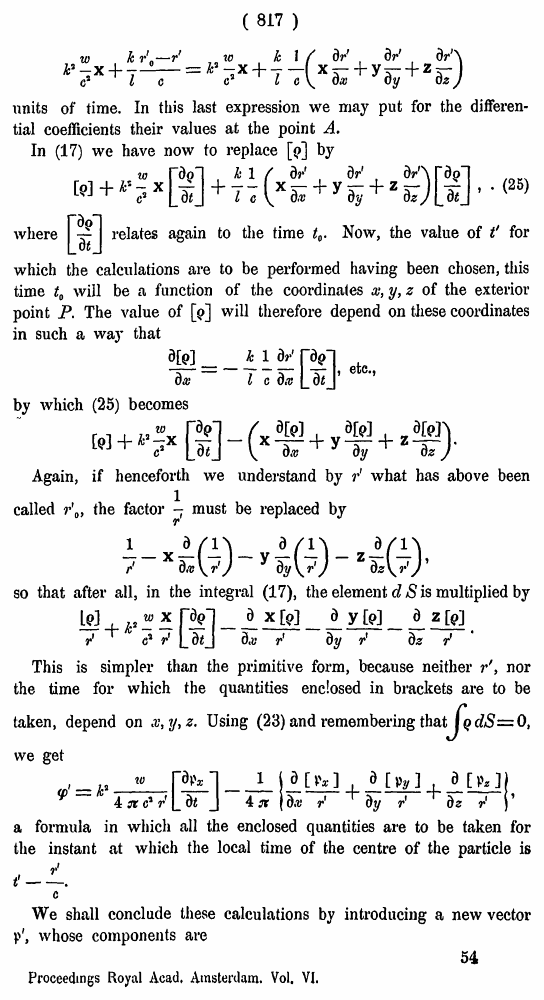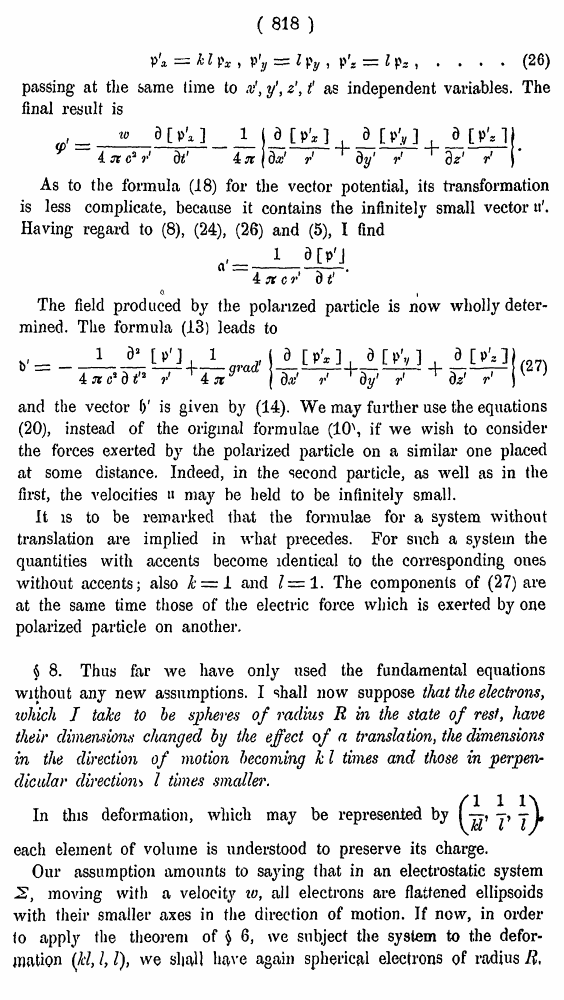# H. A. Lorentz. Electromagnetic phenomena in a system moving with any velocity smaller than that of light. // Proceedings Royal Acad., Amsterdam. Vol. VI., 1904

┬ эрірыю   ─Ёґушх ЄюЁьрҐ√   <<<     ╤ҐЁрэшІр 817   >>>809  810  811  812  813  814  815  816  817 818  819  820  821  822  823  824  825  826  827  828  829  830  831 (817) units of time. In this last expression we may put for the differential coefficients their values at the point A. In (17) we have now to replace [j>] by . Х (25) whererelates again to the time t0. Now, the value of t' for which the calculations are to be performed having been chosen, this time t0 will be a function of the coordinates x, y, z of the exterior point P. The value of  will therefore depend on these coordinates in such a way that etc., by which (25) becomes Again, if henceforth we understand by rС what has above been called r\, the factoimust be replaced by so that after all, in the integral (17), the element cl S is multiplied by This is simpler than the primitive form, because neither r', nor the time for which the quantities enclosed in brackets are to be taken, depend on x, y, z. Using (23) and remembering thai we get a formula in which all the enclosed quantities are to be taken for the instant at which the local time of the centre of the particle is We shall conclude these calculations by introducing a new vector V', whose components are 54 Proceedings Royal Acad, Amsterdam. Vol. VI. ( 818 ) .... (26) passing at the same time to x', y\ z', t as independent variables. The final result is As to the formula (18) for the vector potential, its transformation is less complicate, because it contains the infinitely small vector Having regard to (8), (24), (26) and (5), I find л i The field produced by the polarized particle is now wholly determined. The formula (13) leads to (27) and the vector f)' is given by (14). We may further use the equations (20), instead of the original formulae (10\ if we wish to consider the forces exerted by the polarized particle on a similar one placed at some distance. Indeed, in the second particle, as well as in the first, the velocities ╗ may be held to be infinitely small. It is to be remarked that the formulae for a system without translation are implied in what precedes. For such a system the quantities with accents become identical to the corresponding ones without accents; also k Ч 1 and IЧ 1. The components of (27) are at the same time those of the electric force which is exerted by one polarized particle on another. з 8. Thus far we have only used the fundamental equations without any new assumptions. I shall now suppose that the electrons, which I take to be spheres of radius R in the state of rest, have their dimensions changed by the effect of a translation, the dimensions in the direction of motion becoming k I times and those in perpendicular directionХ> I times smaller. In this deformation, which may be represented by each element of volume is understood to preserve its charge. Our assumption amounts to saying that in an electrostatic system 2, moving with a velocity to, all electrons are flattened ellipsoids with their smaller axes in the direction of motion. If now, in order (o apply the theorem of з 6, we subject the system to the deformation (Jcl, I, I), we sliaU have again spherical electrons of radius B,